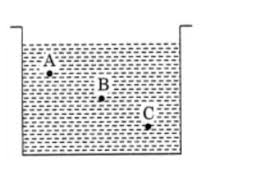(a) Pressure at A > Pressure at B > Pressure at C(b) Pressure at A = Pressure at B = Pressure at C(c) Pressure at A Pressure at C(d) Pressure at A "
">

# A container is filled with water as shown in the given figure. Which of the following statements is correct about pressure of water?(a) Pressure at A > Pressure at B > Pressure at C(b) Pressure at A = Pressure at B = Pressure at C(c) Pressure at A Pressure at C(d) Pressure at A "

The liquid pressure becomes different with the height of the liquid. The pressure inside a liquid at the same depth will be equal.

The pressure of the liquid at depth is directly proportional to the depth (P∝h).

So, option (d) is correct, the Pressure at A< Pressure at B< Pressure at C.

Updated on: 10-Oct-2022

159 Views